# Modulus and argument

Find the mod z and argument z if z=i

m =  1
a =  1.5708

### Step-by-step explanation: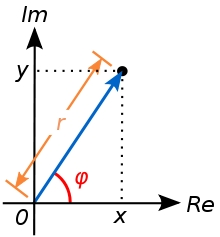Did you find an error or inaccuracy? Feel free to write us. Thank you!Tips to related online calculators
Try our complex numbers calculator.
Most natural application of trigonometry and trigonometric functions is a calculation of the triangles. Common and less common calculations of different types of triangles offers our triangle calculator. Word trigonometry comes from Greek and literally means triangle calculation.

## Related math problems and questions:

• The modulus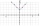Find the modulus of the complex number 2 + 5i
• Linear imaginary equationGiven that 2(z+i)=i(z+i) "this is z star" Find the value of the complex number z.
• Goniometric form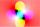Determine the goniometric form of a complex number z = √ 110 +4 i.
• Distance two imaginary numbs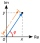Find the distance between two complex number: z1=(-8+i) and z2=(-1+i).
• Log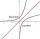Calculate value of expression log |3 +7i +5i2| .
• Complex number coordinatesWhich coordinates show the location of -2+3i
• ABS CNCalculate the absolute value of complex number -15-29i.
• ReciprocalCalculate reciprocal of z=0.8-1.8i:
• Moivre 2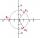Find the cube roots of 125(cos 288° + i sin 288°).
• CotangentIf the angle α is acute, and cotan α = 1/3. Determine the value of sin α, cos α, tg α.
• Cis notationEvaluate multiplication of two complex numbers in cis notation: (6 cis 120°)(4 cis 30°) Write result in cis and Re-Im notation.
• Angle of the body diagonalsUsing vector dot product calculate the angle of the body diagonals of the cube.
• Find the 10Find the value of t if 2tx+5y-6=0 and 5x-4y+8=0 are perpendicular, parallel, what angle does each of the lines make with the x-axis, find the angle between the lines?
• Space vectors 3DThe vectors u = (1; 3; -4), v = (0; 1; 1) are given. Find the size of these vectors, calculate the angle of the vectors, the distance between the vectors.
• Mappings of complex numbersFind the images of the following points under mappings: z=3-2j w=2zj+j-1
• Two boatsTwo boats are located from a height of 150m above the surface of the lake at depth angles of 57° and 39°. Find the distance of both boats if the sighting device and both ships are in a plane perpendicular to the surface of the lake.
• De Moivre's formula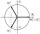There are two distinct complex numbers z, such that z3 is equal to 1 and z is not equal to 1. Calculate the sum of these two numbers.